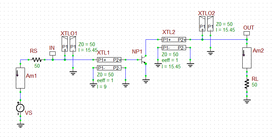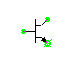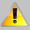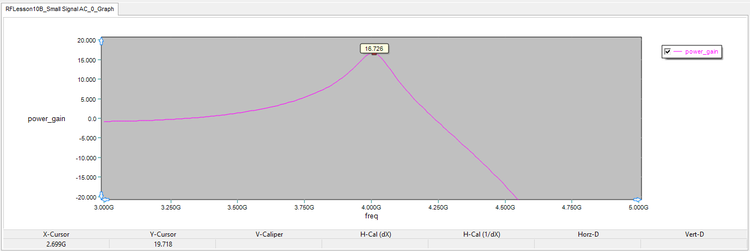# RF Tutorial Lesson 10: Analyzing a Distributed Amplifier Using an Imported RF BJT Model

 Tutorial Project: Analyzing a Distributed Amplifier Using an Imported RF BJT ModelObjective: In this project, you will build and test a distributed RF amplifier using your own S-parameter-based BJT model. Concepts/Features: RF Amplifier S-Parameter-Based BJT Model Maximum Gain Design Tuning Stub Shunt Open Stub Power Gain Minimum Version Required: All versions

## What You Will Learn

In this tutorial you will learn how to import RF BJT models from text files and will build a distributed RF amplifier using a bilateral RF BJT and generic transmission line components.

## Importing High Frequency Transistor Models

RF.Spice's Parts Database contains a sizable collection of RF diodes and BJTs. As you saw in Tutorial Lesson 8, each S-Parameter BJT model corresponds to a certain DC operating point. Therefore, you will find a large number of RF BJT models associated with the same device but measured at different values of VCE and IC. The manufacturer data sheets of RF transistors usually contains a table of measured S-parameter data in the following format:

# GHz   s   ma   r   50

freq1     |s11|     ∠s11     |s21|     ∠s21     |s12|     ∠s12     |s22|     ∠s22

freq2     |s11|     ∠s11     |s21|     ∠s21     |s12|     ∠s12     |s22|     ∠s22

freq3     |s11|     ∠s11     |s21|     ∠s21     |s12|     ∠s12     |s22|     ∠s22

...

You can import text files with a ".TXT" file extension containing S-parameter data of the above format to RF.Spice A/D. Better yet, you can create new active RF devices and add them to expand your parts database. To create a new device, you need to add the following two lines to the header of your text file:

.model <model_name>

.symbol <symbol_name>

The first line creates a unique model name in the database, and the second line picks the right symbol, which is usually either "npn_bjt_2port", or "jfet_n" or "mosfet_n" or "mesfet_n", or their p-type counterparts. For this tutorial lesson, you need to create an RF BJT.

The measured data for the RF BJT device are given below:

f(GHz) s11 s21 s12 s22
3.0 0.80 ∠ -89 ° 2.86 ∠ 99 ° 0.03 ∠ 56 ° 0.76 ∠ -41 °
4.0 0.72 ∠ -116 ° 2.60 ∠ 76 ° 0.03 ∠ 57 ° 0.73 ∠ -54 °
5.0 0.66 ∠ -142 ° 2.39 ∠ 54 ° 0.03 ∠ 62 ° 0.72 ∠ -68 °

Your MyRFBJT.txt file should look like the following:

.model MyRFBJT

.symbol bjt_npn_2port

# GHz   s   ma   r   50

3.0 0.80 -89 2.86 99 0.03 56 0.76 -41

4.0 0.72 -116 2.60 76 0.03 57 0.73 -54

5.0 0.66 -142 2.39 54 0.03 62 0.72 -68

Create a text file as indicated in the table below. Open RF.Spice's Device Manager and select "Create New RF Device from S-Parameter Text File..." from its File Menu. Follow the program's prompts step by step and create your new RF BJT device.

File Name Model Name Symbol Name Symbol
MyRFBJT.txt MyRFBJT bjt_npn_2port## Amplifier Design for Maximum Gain

In this part of the tutorial lesson, you will design an RF amplifier using your MyRFBJT device for maximum gain through conjugate matching. The figure below shows the block diagram of a general transistor amplifier.

The input and output reflection coefficients are given by:

$\Gamma_{in} = \frac{Z_{in} - Z_0}{Z_{in} + Z_0} = s_{11} + \frac{ s_{12}s_{21} \Gamma_L }{1 - s_{22} \Gamma_L}$

$\Gamma_{out} = \frac{Z_{out} - Z_0}{Z_{out} + Z_0} = s_{22} + \frac{ s_{12}s_{21} \Gamma_S }{1 - s_{11} \Gamma_S}$

where ΓS and ΓL are the source and load reflection coefficients as defined in Tutorial Lesson 8.

The maximum power transfer will be achieved under conjugate matching conditions at the input and output of the transistor:

$\Gamma_S^{\ast} = s_{11} + \frac{ s_{12}s_{21} \Gamma_L }{1 - s_{22} \Gamma_L}$

$\Gamma_L^{\ast} = s_{22} + \frac{ s_{12}s_{21} \Gamma_S }{1 - s_{11} \Gamma_S}$

The above equations can be solved for ΓS and ΓL as follows:

$\Gamma_S = \frac{ B_1 \pm \sqrt{B_1^2-4|C_1|^2} }{2C_1}$

$\Gamma_L = \frac{ B_2 \pm \sqrt{B_2^2-4|C_2|^2} }{2C_2}$

where

$B_1 = 1 + |s_{11}|^2 - |s_{22}|^2 - |\Delta |^2$

$B_2 = 1 + |s_{22}|^2 - |s_{11}|^2 - |\Delta |^2$

$C_1 = s_{11} - \Delta s_{22}^{\ast}$

$C_2 = s_{22} - \Delta s_{11}^{\ast}$

$\Delta = s_{11}s_{22} - s_{12}s_{21}$

The maximum transducer gain of the amplifier under the conjugate match conditions is given by:

$G_{Tmax} = G_S . G_0 . G_L = \frac{1}{1-|\Gamma_S|^2} . |S_{21}|^2 . \frac{1-|\Gamma_L|^2}{\left| 1-s_{22} \Gamma_L \right|^2}$

Recalling the definition of the K-parameter from the previous tutorial lesson:

$K = \frac {1 - |s_{11}|^2 - |s_{22}|^2 + |\Delta |^2 } { 2|s_{12}s_{21}| }$

one can show that for an unconditionally stable transistor (K > 1), the maximum transducer gain of the amplifier can be written as:

$G_{Tmax} = \frac{|S_{21}|}{|S_{12}|} \left( K - \sqrt{K^2-1} \right)$

## Building & Testing a Distributed RF BJT Amplifier

The following is a list of parts needed for this part of the tutorial lesson:

Part Name Part Type Part Value
VS AC Voltage Source 1V
NP1 RF NPN BJT Imported Model: MyRFBJT
XTL1 Generic T-Line Z0 = 50, eeff = 1, len = 75
XTL2 Generic T-Line Z0 = 50, eeff = 1, len = 18
XTLO1 Generic Open Stub Z0 = 50, eeff = 1, len = 59.55
XTLO2 Generic Open Stub Z0 = 50, eeff = 1, len = 59.55
RS, RL Resistor 50

The goal is to design a distributed BJT amplifier for maximum gain at f = 4GHz. From the S-parameter data of the RF BJT at 4GHz, you find that

Δ = 0.488 ∠-162°

K = 1.195

ΓS = 0.872 ∠123°

ΓL = 0.876 ∠61°

GTmax = 16.7dB;The property dialog of the imported FR BJT.

The input and output matching networks for this project both consist of a generic 50Ω T-Line segment together with a shunt generic 50Ω T-Line Open Stub as shown in the figure below. The lengths of the T-Line Segments and Open Stubs can be found using the same procedure you followed in Tutorial Lesson 9 for stub matching. Given λ0 = 75mm at f0 4GHz, these lengths are found to be:

T-Line Component Z0 Electrical Length Physical Length at 4GHz
T1 50Ω 0.120λ0 9mm
XTLO1 50Ω 0.206λ0 15.45mm
T2 50Ω 0.206λ0 15.45mm
XTLO2 50Ω 0.206λ0 15.45mm

Place and connect all the parts as shown in the figure below. Place a net marker called "IN" before the input open stub and place another net marker called "OUT" after the output open stub.The distributed RF BJT Amplifier without the source and load sections for the purpose of network analysis.

Run a Network Analysis Test of your circuit with the parameters specified below:

 Start Frequency 3G 5G 10Meg Linear S Smith or Cartesian (Amplitude Only) with Decibels checked

The figure below shows the results for S11, S21, S12 and S22 parameters. It can be seen that the amplifier has a reasonably good return loss at 4GHz. Note that your original S-parameter model of the RF BJT has data for only thee frequencies. Using crosshairs you can also read the insertion gain |s21| to be 16.7dB as was the design goal.RF.Spice A/D interpolates between a model's available frequency data points to calculate the S-parameters at all the intermediate frequencies. Therefore, a larger number of measured frequency data points leads to more accurate simulation results.The graph of magnitude of S11, S21, S12 and S22 parameters of the distributed BJT amplifier circuit.

## Running an AC Frequency Sweep to Compute Power Gain

Next, connect the AC voltage source and the source and load resistors and place two ammeters at the source and load ammeters in a similar manner as in the last part of Tutorial Lesson 8. The figure below shows the circuit with the source, load and ammeters:The distributed RF BJT Amplifier with generic T-Line components.

Similarly, define a custom output plot called "Power_Gain" for your amplifier. Use the same definition: GP = 20*log10(abs(i(am2)/i(am1))). Run an AC Frequency Sweep Test of your amplifier according to the table below:

 Start Frequency 3G 5G 10Meg Linear Custom: Power_Gain

The figure below shows the graph of power gain vs. frequency, where you can see the maximum value found to be 16.726dB. This agrees perfectly with you computed insertion gain in the previous part.The graph of the power gain of the distributed BJT amplifier vs. frequency.